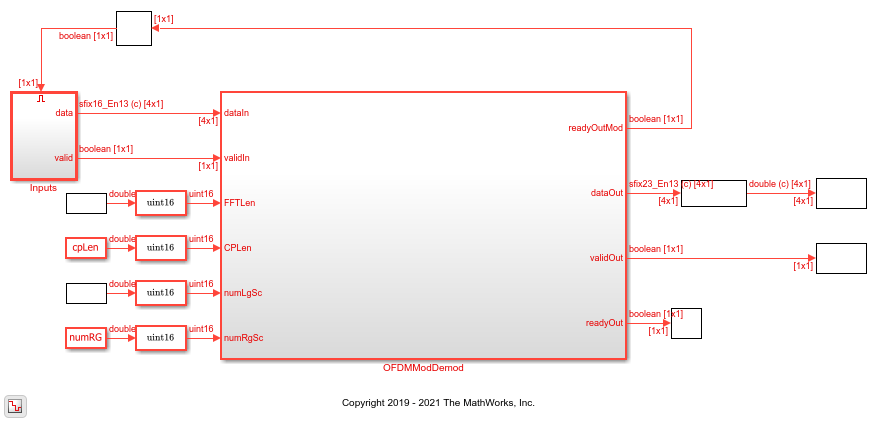# Modulate and Demodulate OFDM Streaming Samples

This example model shows how to use OFDM Modulator and OFDM Demodulator blocks. In this model, an OFDM Modulator and an OFDM Demodulator block are connected back-to-back. The OFDM parameters source parameter in these blocks is set to `Input port`, enabling you to dynamically change the input values of these blocks. You can change these values using the script in this example. These blocks support scalar and vector inputs. To verify the functionality of these blocks, the input provided to the OFDM Modulator block is compared with the output of the OFDM Demodulator block. The `OFDMModDemod` HDL subsystem in this example model supports HDL code generation.

### Set Input Data Parameters

Set up these workspace variables for the model to use. You can modify these values according to your requirement. The example model uses these workspace variables `dataIn`, `validIn`, `fftLen`, `maxFFTLen`, `cpLen`, `numLG`, `numRG`, `numSymb`, and `DCNull` to configure the OFDM Modulator and OFDM Demodulator blocks.

```fftLen = 64; maxFFTLen = 128; cpLen = 16; numLG = 6; numRG = 5; numSymb = 2; DCNull = 1; % 1 or 0 vecLen = 4; % 1, 2, 4, 8, 16, 32, or 64 if DCNull==1 numActData = fftLen - (numLG+numRG+1); else numActData = fftLen - (numLG+numRG); end ```

### Generate Input Data Frames

Generate random frames of complex input data and a control signal that indicates the frame boundaries.

```rng default; dataIn = complex(randn(numActData*numSymb,1),randn(numActData*numSymb,1)); dataVec = []; % Store data arranged in vector form presentSymbDataStartIndex = 0; for ii = 1:numSymb counter = 0; for jj = 1:ceil(numActData/vecLen) if jj == ceil(numActData/vecLen) numZerosTobeAppended = vecLen - (numActData-counter); dataVec = [dataVec [dataIn(presentSymbDataStartIndex+counter+(1:vecLen-numZerosTobeAppended));zeros(1,numZerosTobeAppended).']]; else dataVec = [dataVec dataIn(presentSymbDataStartIndex+counter+(1:vecLen))]; end counter = counter + vecLen; end presentSymbDataStartIndex = presentSymbDataStartIndex + numActData; end data = dataVec.'; valid = boolean(ones(size(data,1),1)); % Valid signal generation sampling_time = 1; stoptime = maxFFTLen*6*numSymb; ```

Run the model to import the input signal variables `dataIn`, `validIn`, `fftLen`, `maxFFTLen`, `cpLen`, `numLG`, `numRG`, `numSymb`, and `DCNull` from the workspace to the OFDM Modulator block. The OFDM Modulator block returns OFDM-modulated output samples and a control signal. These OFDM-modulated samples are fed to the OFDM Demodulator block, which returns OFDM demodulated samples.

```open_system('genhdlOFDMModDemodExample') sim('genhdlOFDMModDemodExample'); % Store valid data from Simulink model dataOut1 = dataOut.data; simOut = dataOut1(:,:,validOut); simOut = simOut(:); ```### Compare OFDM Modulator Input with OFDM Demodulator Output

Compare the input data provided to the OFDM Modulator block with the output data generated from the OFDM Demodulator block.

```figure('units','normalized','outerposition',[0 0 1 1]) subplot(2,1,1); plot(real(dataIn(1:size(simOut)))); hold on plot(squeeze(real(simOut))); legend('Real part of reference data','Real part of demodulated data'); title('Comparison of OFDM Modulator Input with OFDM Demodulator Output - Real Part'); xlabel('OFDM Subcarriers'); ylabel('Real Part'); subplot(2,1,2) plot(imag(dataIn(1:size(simOut)))); hold on plot(squeeze(imag(simOut))) legend('Imaginary part of reference data','Imaginary part of demodulated data'); title('Comparison of OFDM Modulator Input with OFDM Demodulator Output - Imaginary Part'); xlabel('OFDM Subcarriers'); ylabel('Imaginary Part'); ```